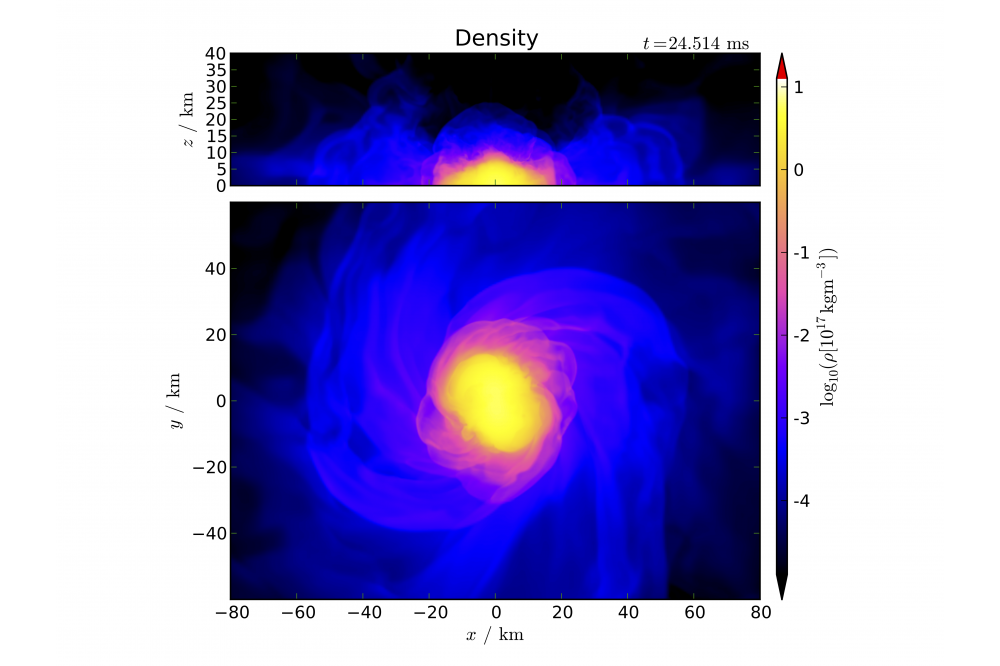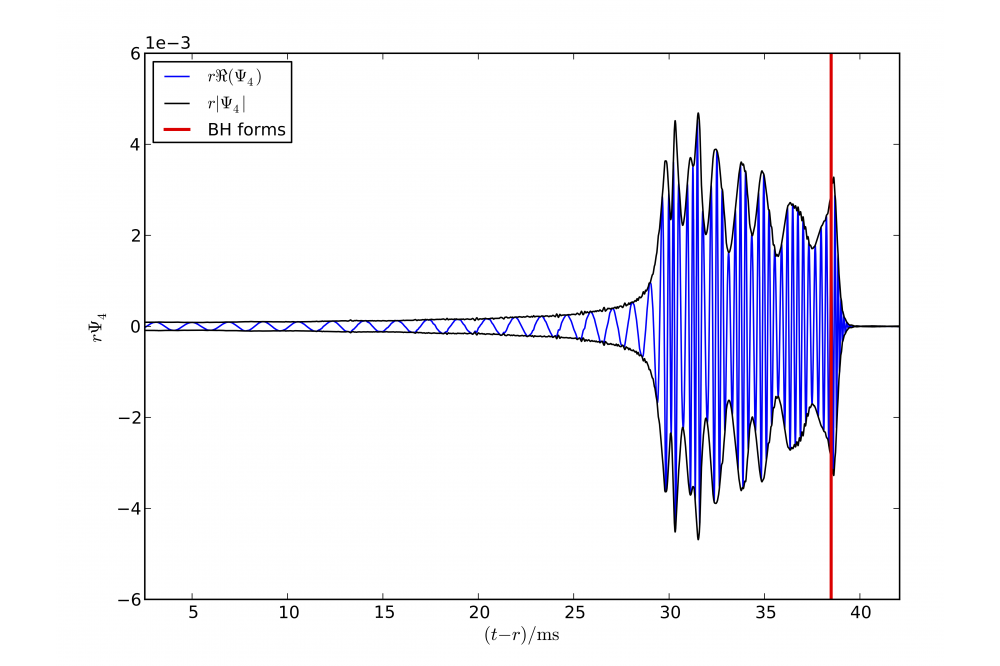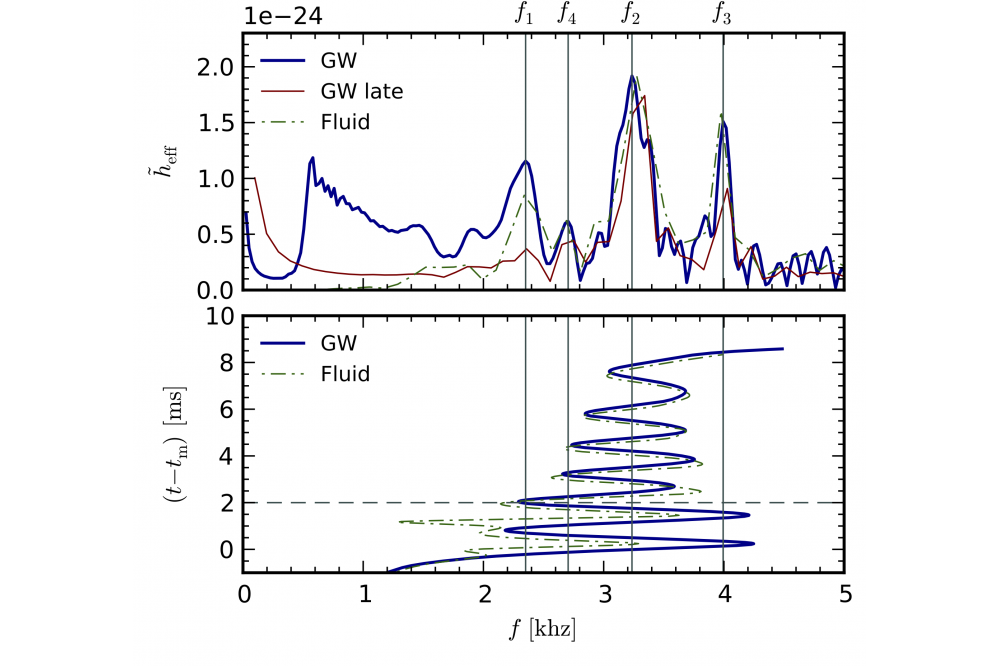# On the Properties of Hypermassive Neutron Stars Formed in Mergers of Spinning BinariesHypermassive Neutron Stars 1

Fig. 1: Snapshot of a merger simulation of two NS with 2 solar masses each, assuming the Shen-Horowitz-Teige EOS. The NS spins are aligned to the orbital angular momentum. Color-coded is the mass density in the orbital plane (lower panel) and the x-z-plane (upper panel).Hypermassive Neutron Stars 2

Fig. 2: Gravitational wave signal of a merger of two NS with 1.5 solar masses each, assuming the Lattimer-Swesty EOS. The first part of the signal is caused the inspiral phase, the next stage is due to a hypermassive neutron star created in the merger, which then collapses to a BH (vertical line) responsible for the remaining signal (ring-down).Hypermassive Neutron Stars 3

Fig. 3: (Top panel) Gravitational wave effective strain spectrum the gravitational signal shown in Figure 2. (Bottom panel) Evolution of the instantaneous frequencies. The instantaneous gravitational wave frequency is strongly modulated during the HMNS stage.

## Introduction

Binary neutron star (BNS) mergers are among the most promising sources of gravitational waves (GW). In this project, we performed three dimensional, fully relativistic numerical simulations of coalescing binary neutron star systems with different spins and two different nuclear equations of state (EOS).

## Methods

The outcome of BNS mergers is mainly determined by the masses and spins of the two neutron stars (NS), and by the behaviour of matter at high densities, as described by EOS. If the mass is above some threshold, the two stars promptly collapse to a black hole (BH) upon merger. Below the threshold, they form a metastable or even stable NS. The threshold depends strongly on the EOS and on the spin. The case where a hypermassive neutron (HMNS) star is formed is particularly interesting since it emits a strong GW signal which carries information about the EOS. By varying the spin we determined its influence on the GW signal and the rotation profile of the HMNS. Figure 1 displays a snapshot of the density profile showing an HMNS formed during the merger. In the GW signal (see Figure 2) we can observe a modulation during the HMNS stage induced by strong quasi-radial oscillation. Figure 3 shows an example for the time-dependent GW frequency spectrum, from the increasing orbital frequency during the inspiral, over the rich spectrum of the HMNS stage, to the formation of a BH.

## Last Update

• Last Update: 2020-11-12 18:13